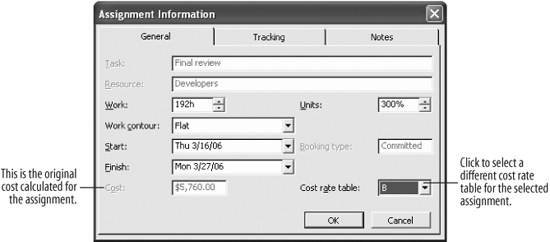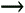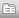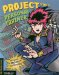# Lesson7.4.Applying a Different Pay Rate to an Assignment

### Lesson 7.4. Applying a Different Pay Rate to an Assignment

##### Figure 7-4. The Assignment Information dialog boxIn the previous lesson, you learned how to add pay rates to a resource. This lesson will show you how to apply one of these additional pay rates to a resource assignment.

1. Select ViewThe resources in the project appear.

2. Scroll down to the Developers resource.

This resource has a number of assignments. Currently, all work is being done at the same pay rate. However, the resource's contract specifies a different pay rate for the "Final review" task.

3. Select the Final Review task under the Developers resource. Click theAssignment Information button on the Standard toolbar.

The General tab of the Assignment Information dialog box appears, as shown in Figure 7-4.

The current cost calculation of the task is \$5,760. This will change with a new cost rate table.

4. Click the Cost rate table list arrow and select B.

This is the pay rate table you added in the previous lesson. This pay rate is less than the original (table A), so the total cost of the assignment will drop.

5. Click OK.

The dialog box closes. Note that the calculated cost has changed: the cost with the new rate is \$4,416.

## TO APPLY A DIFFERENT PAY RATE TO AN ASSIGNMENT:

1. Select ViewSelect the assignment you want to change.

2. Click the Assignment Information button on the Standard toolbar.

3. Click the Cost rate table list arrow and select the table you want to use.

4. Click OK.Project 2003 Personal Trainer
ISBN: 0596008546
EAN: 2147483647
Year: 2005
Pages: 156

Similar book on Amazon